# Linear connection

This lives as an element of: the space of$\R$-bilinear maps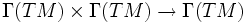$\Gamma(TM) \times \Gamma(TM) \to \Gamma(TM)$

## Definition

### Given data

• A connected differential manifold$M$ with tangent bundle denoted by$TM$

### Definition part (pointwise form)

A linear connection is a smooth choice$\nabla$ of the following: at each point$p \in M$, there is a map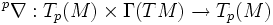${}^p\nabla: T_p(M) \times \Gamma(TM) \to T_p(M)$, satisfying some conditions. The map is written as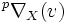${}^p\nabla_X(v)$ where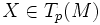$X \in T_p(M)$ and$v \in \Gamma(TM)$.

• It is$\R$-linear in$X$ (that is, in the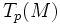$T_p(M)$ coordinate).
• It is$\R$-linear in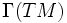$\Gamma(TM)$ (viz the space of sections on$TM$).
• It satisfies the following relation called the Leibniz rule: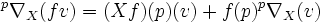${}^p\nabla_X(fv) = (Xf)(p) (v) + f(p) ^p\nabla_X(v)$

### Definition part (global form)

A linear connection is a map$\nabla:\Gamma(TM) \times \Gamma(TM) \to \Gamma(TM)$, satisfying the following:

• It is$C^\infty$-linear in the first$\Gamma(TM)$
• it is$\mathbb{R}$-linear in the second$\Gamma(TM)$
• It satisfies the following relation called the Leibniz rule: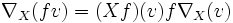$\nabla_X(fv) = (Xf) (v) f \nabla_X(v)$

where$f$ is a scalar function on the manifold and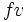$fv$ denotes scalar multiplication of$v$ by$f$.

### Generalizations

The notion of linear connection can be generalized to the more general notion of a connection, where the second$TM$ is replaced by an arbitrary vector bundle$E$ over$M$.

## Operations on a linear connection

### Torsion of a linear connection

Further information: torsion of a linear connection

The torsion of a linear connection$\nabla$ is denoted as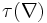$\tau(\nabla)$. It is a$(1,2)$-tensor defined as: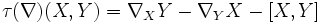$\tau(\nabla)(X,Y)= \nabla_XY - \nabla_YX - [X,Y]$.

A connection whose torsion is zero is termed a torsion-free linear connection.

Note that torsion makes sense only for linear connections.

### Curvature of a linear connection

Further information: Riemann curvature tensor

The curvature of a linear connection$\nabla$ is denoted as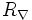$R_\nabla$. It is defined as: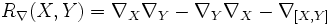$R_\nabla(X,Y) = \nabla_X\nabla_Y - \nabla_Y\nabla_X - \nabla_{[X,Y]}$

The notion of curvature actually makes sense for any connection.